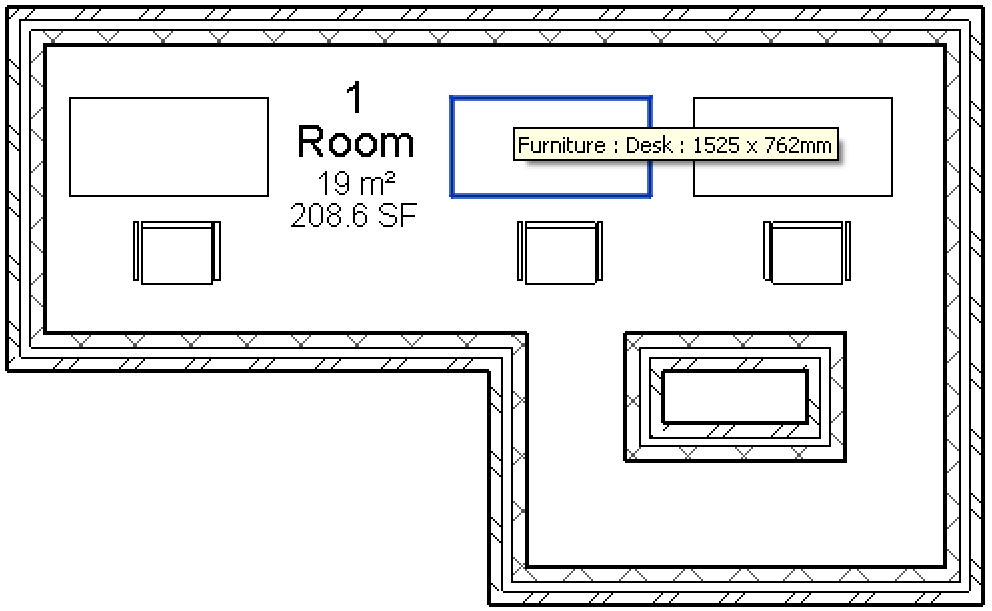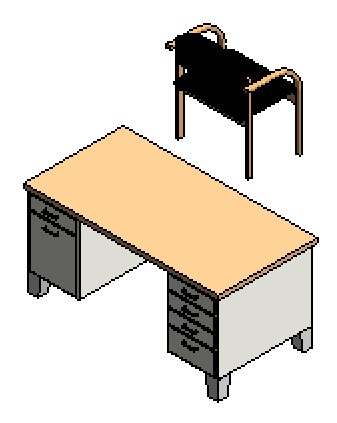### Sort and Orient Curves to Form a Contiguous Loop

Continuing the research and development for my cloud-based round-trip 2D Revit model editing project, I need to determine the boundary loop polygons to represent the furniture and equipment family instances for manipulation on the mobile device.

To display a polygon using SVG in the mobile device browser, I obviously need a set of contiguous and sorted curve elements forming a closed loop.

I mentioned using the Revit API ExtrusionAnalyzer class to determine the plan view boundary outline for the family instances. On testing that approach, I discovered that the results it returns are unsorted.

For instance, I analysed the ExtrusionAnalyzer output for the standard Revit furniture content 'Desk 1525 x 762mm'. In plan view, it can appear like this:The desk includes lots of internal geometry that is not visible in plan view:As a result, the information returned by the ExtrusionAnalyzer is much more complex than the simple rectangle one might expect. In fact, it returns ten separate closed loops, and the first one consists of eight curves.

To check whether they are contiguous and correctly sorted, here is a list of their end points:

```  (2.74,8.38,0) --> (2.74,8.46,0)
(2.74,8.38,0) --> (2.76,8.38,0)
(2.76,8.38,0) --> (2.76,8.44,0)
(2.76,8.44,0) --> (3.05,8.44,0)
(3.05,8.44,0) --> (3.05,8.38,0)
(3.05,8.38,0) --> (3.08,8.38,0)
(3.08,8.46,0) --> (3.08,8.38,0)
(2.74,8.46,0) --> (3.08,8.46,0)
```

You can see quite easily that they are not contiguous. For instance, the end point at (2.74,8.46) of the first curve equals the start point of the last.

If you look more carefully still, you will notice that some of the curves require reversing to connect to their neighbours.

These observations and considerations led to the implementation of the following curve sorting and orientation method.

Its end point matching comparison relies on the standard Revit precision, which is around one sixteenth of an inch. Since the built-in Revit database length unit is feet, I define the following fuzz factor for that:

```  const double _inch = 1.0 / 12.0;
const double _sixteenth = _inch / 16.0;
```

Further, the curve reversal is implemented by creating a completely new curve. Therefore, the creation application has to be provided for generating these:

```/// <summary>
/// Create a new curve with the same
/// geometry in the reverse direction.
/// </summary>
/// <param name="orig">The original curve.</param>
/// <returns>The reversed curve.</returns>
/// <throws cref="NotImplementedException">If the
/// curve type is not supported by this utility.</throws>
static Curve CreateReversedCurve(
Autodesk.Revit.Creation.Application creapp,
Curve orig )
{
if( !IsSupported( orig ) )
{
throw new NotImplementedException(
"CreateReversedCurve for type "
+ orig.GetType().Name );
}

if( orig is Line )
{
return creapp.NewLineBound(
orig.GetEndPoint( 1 ),
orig.GetEndPoint( 0 ) );
}
else if( orig is Arc )
{
return creapp.NewArc( orig.GetEndPoint( 1 ),
orig.GetEndPoint( 0 ),
orig.Evaluate( 0.5, true ) );
}
else
{
throw new Exception(
"CreateReversedCurve - Unreachable" );
}
}
```

With this support in hand, we can go ahead and implement the SortCurvesContiguous method:

```/// <summary>
/// Sort a list of curves to make them correctly
/// ordered and oriented to form a closed loop.
/// </summary>
public static void SortCurvesContiguous(
Autodesk.Revit.Creation.Application creapp,
IList<Curve> curves,
bool debug_output )
{
int n = curves.Count;

// Walk through each curve (after the first)
// to match up the curves in order

for( int i = 0; i < n; ++i )
{
Curve curve = curves[i];
XYZ endPoint = curve.GetEndPoint( 1 );

if( debug_output )
{
Debug.Print( "{0} endPoint {1}", i,
Util.PointString( endPoint ) );
}

XYZ p;

// Find curve with start point = end point

bool found = (i + 1 >= n);

for( int j = i + 1; j < n; ++j )
{
p = curves[j].GetEndPoint( 0 );

// If there is a match end->start,
// this is the next curve

if( _sixteenth > p.DistanceTo( endPoint ) )
{
if( debug_output )
{
Debug.Print(
"{0} start point, swap with {1}",
j, i + 1 );
}

if( i + 1 != j )
{
Curve tmp = curves[i + 1];
curves[i + 1] = curves[j];
curves[j] = tmp;
}
found = true;
break;
}

p = curves[j].GetEndPoint( 1 );

// If there is a match end->end,
// reverse the next curve

if( _sixteenth > p.DistanceTo( endPoint ) )
{
if( i + 1 == j )
{
if( debug_output )
{
Debug.Print(
"{0} end point, reverse {1}",
j, i + 1 );
}

curves[i + 1] = CreateReversedCurve(
creapp, curves[j] );
}
else
{
if( debug_output )
{
Debug.Print(
"{0} end point, swap with reverse {1}",
j, i + 1 );
}

Curve tmp = curves[i + 1];
curves[i + 1] = CreateReversedCurve(
creapp, curves[j] );
curves[j] = tmp;
}
found = true;
break;
}
}
if( !found )
{
throw new Exception( "SortCurvesContiguous:"
+ " non-contiguous input curves" );
}
}
}
```

This is obviously not the most effective sorting algorithm in the world, but it should do for the hopefully simple cases I expect to encounter.

This method includes an option for verbose logging to the Visual Studio debug output window. This is what it produces for the unsorted list of input points provided above:

```  0 endPoint (2.74,8.46,0)
7 start point, swap with 1
1 endPoint (3.08,8.46,0)
6 start point, swap with 2
2 endPoint (3.08,8.38,0)
5 end point, swap with reverse 3
3 endPoint (3.05,8.38,0)
4 end point, reverse 4
4 endPoint (3.05,8.44,0)
5 end point, reverse 5
5 endPoint (2.76,8.44,0)
6 end point, reverse 6
6 endPoint (2.76,8.38,0)
7 end point, reverse 7
7 endPoint (2.74,8.38,0)
```

The complete output for the entire desk looks like this after sorting and rearranging all ten loops using the SortCurvesContiguous method and converting the results from Revit XYZ coordinates to my 2D integer-based loop representation in millimetres:

```FamilyInstance Furniture Desk <212646 1525 x 762mm> has 10 loops:
0: (836,2555), (836,2580), (937,2580), (937,2555), (931,2555), (931,2574), (842,2574), (842,2555)
1: (1954,2580), (1954,2555), (1961,2555), (1961,2574), (2050,2574), (2050,2555), (2056,2555), (2056,2580)
2: (683,2542), (683,1780), (2208,1780), (2208,2542), (1802,2542), (1802,1831), (1090,1831), (1090,2542)
3: (664,2561), (664,1761), (2227,1761), (2227,2561)
4: (683,2440), (785,2440), (785,2542), (683,2542)
5: (785,1780), (785,1882), (683,1882), (683,1780)
6: (2107,1882), (2107,1780), (2208,1780), (2208,1882)
7: (2107,2542), (2107,2440), (2208,2440), (2208,2542)
8: (702,2542), (702,2555), (1071,2555), (1071,2542)
9: (1821,2542), (1821,2555), (2189,2555), (2189,2542)
```

I hope you can make good use of this method in your own add-ins as well.

Happy Easter!